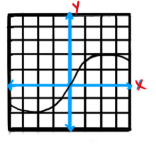# Use the given graph to estimate the value of each derivative

Use the given graph to estimate the value of each derivative. Then sketch the graph of f’(3).
Given graph